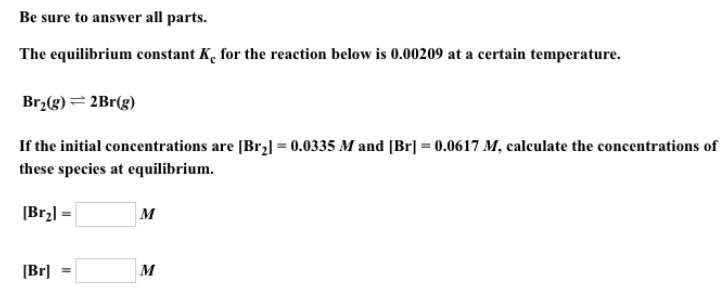# Problem: Be sure to answer all parts.The equilibrium constant Kc for the reaction below is 0.00209 at a certain temperature. Br2 (g) ⇌ 2Br (g) If the initial concentrations are [Br2] = 0.0335 M and [Br] = 0.0617 M, calculate the concentrations of these species at equilibrium. [Br2] = [Br] =

###### FREE Expert Solution
92% (219 ratings)###### Problem Details

Be sure to answer all parts.

The equilibrium constant Kc for the reaction below is 0.00209 at a certain temperature.

Br2 (g) ⇌ 2Br (g)

If the initial concentrations are [Br2] = 0.0335 M and [Br] = 0.0617 M, calculate the concentrations of these species at equilibrium.

[Br2] =

[Br] =